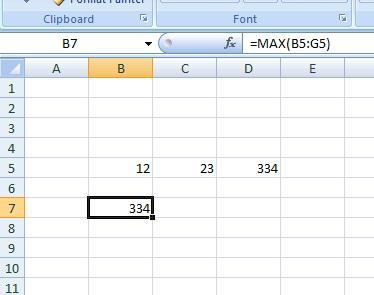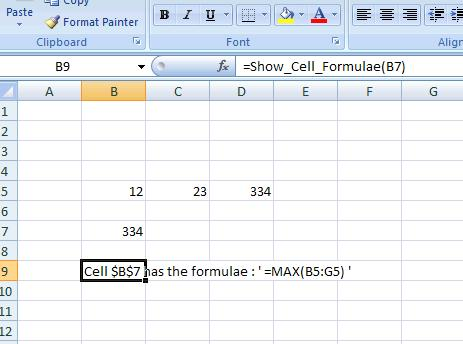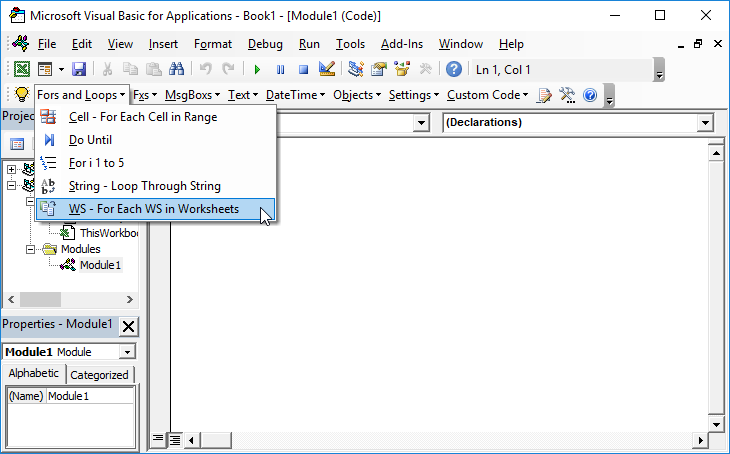# VBA Function to Show Cell Formula

The following UDF will return the formulae for a given cell reference:

## VBA Function to Show Cell Formula

``````Function Show_Cell_Formulae(Cell As Range) As String
Show_Cell_Formulae = "Cell " & Cell.Address & " has the formulae: " & Cell.Formula & " '"
End Function``````

So if we have:Where the cell B7 has the formulae : ‘=MAX(B5:G5) then we can type in another cell:
B9 = Show_Cell_Formulae(b7)And we get a text string with the cell reference and the formulae contained within it.